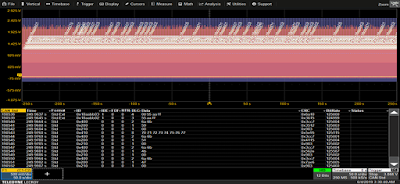## You need to test, we're here to help.

You need to test, we're here to help.

## 01 March 2021

### TDME Primer: Selecting Sample Rate for Serial Bus AnalysisFigure 1. Sample rate of only four sample points per bit decodes correctly and lengthens serial bus acquisition.
Teledyne LeCroy supports trigger, decode, measure/graph, and eye diagram (TDME) software options for over 20 serial data standards, and the list is growing. This series will address practical tips for using TDME software successfully, and showcase some examples of applying TDME capabilities to real-world problems.

Given the wide range of protocols supported, you might be curious about how to best choose the oscilloscope sampling rate for a given standard when acquiring serial data signals. The optimal sample rate is determined by three principal factors:

1) the bandwidth of the signal being digitized by the oscilloscope’s analog-to-digital converter (ADC);

2) the desired duration of the acquisition;

3) what you are going to do with the acquisition.

You don't usually hear about point three when discussing sample rate, but it can actually be a significant factor in your selection.

The signal bandwidth identifies the highest frequency to be digitized by the ADC. The Nyquist criteria requires that the sample rate should be twice the highest frequency. The bandwidth of a digital signal, such as encountered in serial data analysis, can be estimated by knowing the risetime of an acquired digital edge from the equation:

BW =  k/Trise

Where the bandwidth (BW) in Hertz is the ratio of a factor k to the signal risetime (Trise) in seconds.

The value k depends on the frequency response of the oscilloscope.  For a single pole response, typical in oscilloscopes with bandwidths below 1 GHz, k = 0.35.  For oscilloscopes with a brick wall response, typically those with bandwidths of 1 GHz or higher, the factor k = 0.45.

For example, consider a CAN signal running at a bit rate of 125 kilobits per second (kb/s) with a risetime of approximately 21 ns. If the oscilloscope has a single pole response, the bandwidth of the acquired signal is 16.7 MHz.  The Nyquist frequency is 33.4 MHz.  This is the minimum sample rate required to digitize a signal with a 16.7 MHz bandwidth. For most serial data applications, it is advisable to oversample by a factor of three or four times the Nyquist frequency to assure the most accurate physical layer measurements.

However, decoding the bus does not require as high a sample rate as does making accurate physical layer measurements. In fact, for lower-speed protocols, the sample rate can be reduced to as little as four samples points per bit and still retain the ability to accurately decode the packet data content. If all you need to do is perform basic measurements on packet timing, validate correct transitions, find protocol errors or other tasks that can be accomplished by a visual assessment of bus traffic, you can buy valuable acquisition time by significantly lowering the sample rate.

In Figure 1 above, the CAN signal is sampled at four times the 125 kb/s clock rate, or 500 kS/s. The zoom in the middle of the figure clearly shows the four sample points in each clock period. This sample rate is sufficient to characterize the logic states and decode the data for an initial visual assessment of the traffic.

The next consideration is the desired duration of the acquisition to be analyzed. This is determined by the length of the oscilloscope memory:

T = M/SR

Where T is the acquisition duration, in seconds, M is the memory length in number of samples, and SR is the sample rate in samples per second (S/s).Figure 2: 125 kb/s CAN signal sampled at 500 kS/s on a 250 MS memory oscilloscope collects 500 S of data amounting to 190,552 messages.
A longer acquisition time may be required in order to assess characteristics like bus loading, the percentage of time the bus is active. If we use a clock rate of 500 kS/s with an oscilloscope that has a 250 MS memory length, then using the equation above, the record length is 500 seconds or 8.3 minutes of data.  Figure 2 shows an acquisition of 190,552 CAN message packets at this sampling rate. That is quite a bit of data.

On the other hand, a higher sample rate provides more accurate physical layer measurements. If you need to measure the electrical characteristics of a signal, sample at the highest rate that will allow you to collect a long enough acquisition to fully show what you need to measure. Remember: many measurements are only made on the visible acquisition window, so be sure you've actually captured what you need (our next post will provide some tips for that).Figure 3: Impact of sample rate on 125 kb/s CAN signal risetime measurements.
As Figure 3 shows, at a sample rate:clock rate ratio of 800:1, the risetime measurement error can be as much as 5% of the true value. Below that sample rate, the edges are insufficiently sampled to render a correct measurement.

When choosing the oscilloscope sample rate (SR) for serial bus analysis, a good general rule of thumb is:

• Decoding: SR at least four times the clock rate
• Eye diagrams: SR at least 100 times the clock rate
• Physical layer measurements: SR at least 1000 times the clock rate

For more on this topic, see our on-demand webinar, Measuring and Debugging CAN and CAN FD. Much of the information in it is relevant to any protocol.